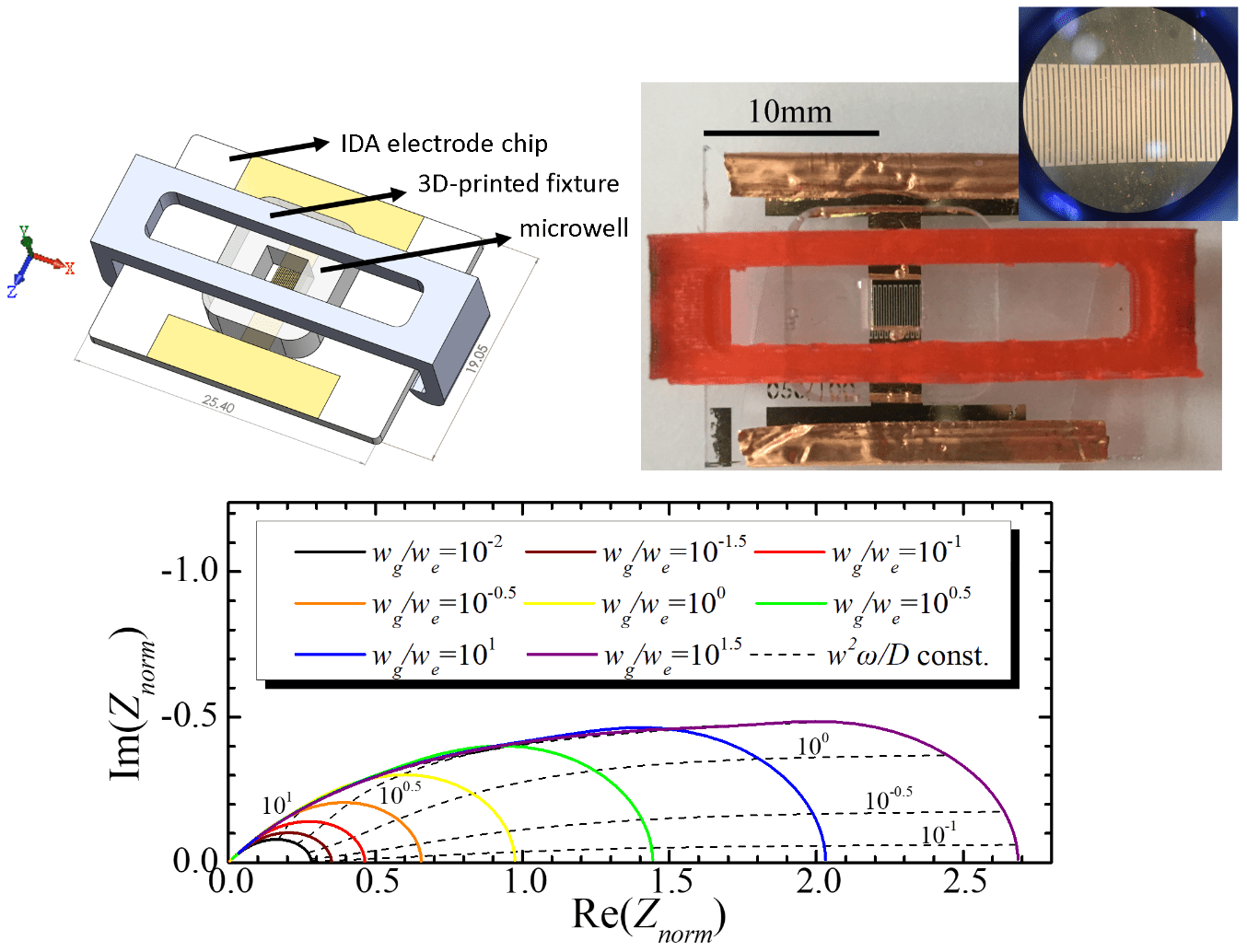# Diffusion Impedance Modeling of Interdigitated Array Electrodes

While I was working with interdigitated array electrodes (IDA electrodes) for my previous research Real-time Impedimetric MUC1 Aptasensor using Microfluidic Symmetric Gold Electrode, I discovered a major problem with its impedance spectrum when viewing the Nyquist plot. Until then, there hadn’t been any studies that can explain the diffusion phenomenon of redox species between the band electrodes due to an applied sinusoidal voltage wave, and no circuit elements appear to be suitable for modeling the diffusion impedance of IDA electrodes. Therefore, I devised a theory for modeling an IDA electrodes impedimetric properties using conformal mapping and cylindrical finite length approximation methods, and succeeded to construct a circuit element that can replace the Warburg element, and extract important parameters from the corresponding equivalent circuit model. This can aid researchers in relevant fields to model their systems more accurately.

Here’s a video for visualizing the diffusion phenomenon between the band electrodes of an IDA electrode. COMSOL is used for simulating the time-dependent concentration field of redox species.

Abstract

An analytical problem for impedimetric sensing is usually encountered when using interdigitated array (IDA) electrodes. Finite diffusion of redox species dominates at low frequencies and confuses researchers, making incorrect understanding of underlying phenomena possible. In this work, an integral equation for calculating the diffusion impedance of IDA electrodes is derived using conformal mapping and cylindrical finite length approximation. Electrodes of different bandwidths and gap widths are fabricated, and their heights and symmetric electrochemical characteristics are verified. Simulations are performed to verify the predicted constant concentration contours. The calculated zero-frequency impedance showed high correlation with the reciprocal of limiting current calculated from literature study (R2 = 0.992) and from chronoamperometry experiments (R2 = 0.970). Further evidence for the correctness of theory is established due to the fact that experimental EIS data and calculated impedances are highly consistent (R2 ≥ 0.948 for real and imaginary part). This sheds some light on explaining the diffusion phenomenon of impedance using IDA electrodes in the low frequency spectrum. An equivalent circuit fitting program is further designed for fitting several elements including the IDA electrode diffusion impedance derived in the theory. The program succeeded to accurately fit the EIS data (average MSE = 0.611), which using the Warburg element failed (average MSE = 54.86). Parameters such as the ratio of electrode bandwidth to gap width and diffusion coefficient can also be determined by fitting the data from a single EIS experiment. Another impedance calculation program is also given, which can aid researchers in relevant fields to model their systems more accurately.

Related Publications

1. C.-Y. Lai, J.-H. Weng, W.-L. Shih, L.-C. Chen, C.-F. Chou, P.-K. Wei, Diffusion impedance modeling for interdigitated array electrodes by conformal mapping and cylindrical finite length approximation, Electrochimica Acta, 320 (2019) 134629.
https://doi.org/10.1016/j.electacta.2019.134629
2. C.-Y. Lai, J.-H. Weng, W.-L. Shih, L.-C. Chen, C.-F. Chou, P.-K. Wei, Diffusion impedance modeling for interdigitated array electrodes by conformal mapping and cylindrical finite length approximation, 11th International Symposia on Electrochemical Impedance Spectroscopy, (2019).
[abstract] [presentation pdf] [presentation clip]